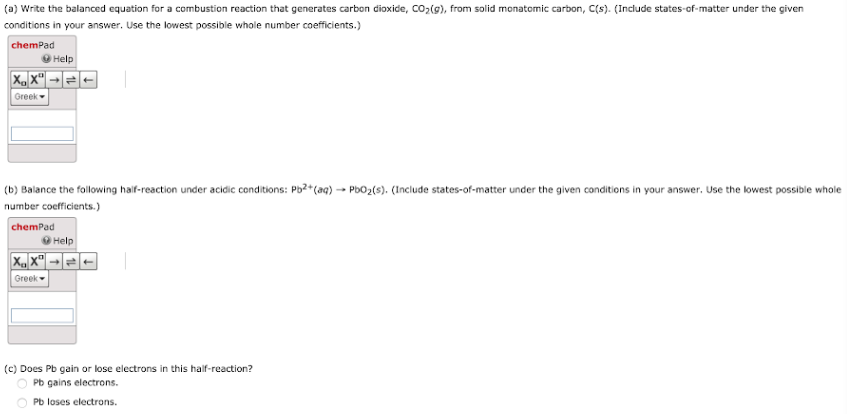# (a) Write a balanced equation for a combustion reaction that generates carbon dioxide, CO2(g), from solid monatomic carbon, C(s). (Include states-of-matter under the given conditions in your answer, Use the lowest possible whole number coefficients.) (b) Balance the following half-reaction under acidic conditions: Pb2+(aq) -> PbO2(s). (Include states-of-matter under the given conditions in your answer. Use the lowest possible whole number coefficients.) (c) Does Pb gain or lose electrons in this half-reaction?